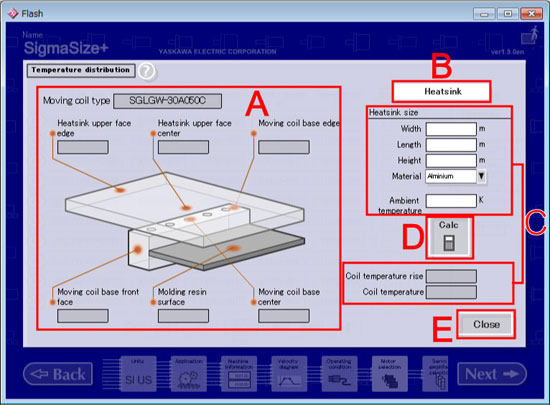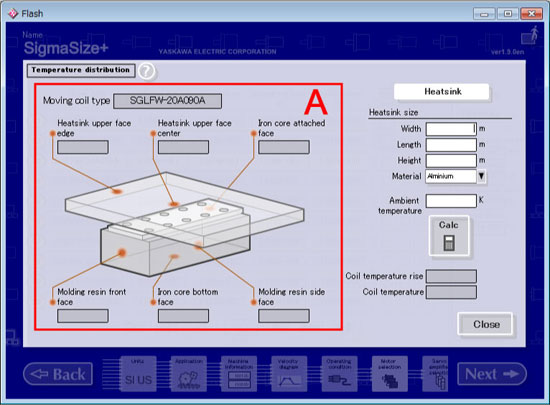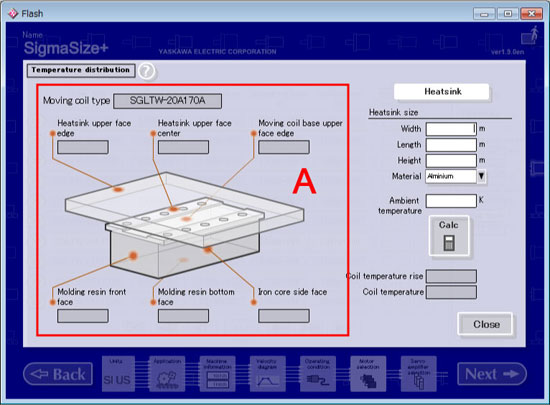Wizard sizing / Temperature distribution

Temperature distribution(only for the Linear Type)

Calculates temperature distribution for a moving coil and heat sink for the linear type.
There are SGLGW / SGLFW / SGLTW to moving coils, their shape and features are different.

[Operation Procedure]

1. Decide whether calculate the temperature of a heat sink.
• If attach
1. Turn the "Heat sink" button ON.
2. Input the size of heat sink and a ambient temperature.
• If not attach
1. Turn the "Heat sink" button OFF.
2. Input a ambient temperature.
2. Click "Calc"
Calculates and displays winding temperature rise, and each part temperature.
When the material of a heat sink is iron, assume an about 1.5 times as much temperature rise as the value calculated with alminium.

[Screen Structure]Temperature distribution Screen (Moving Coil: SGLGW)

1. Moving Coil Diagram
The next calculation result is displayed.

• Heat sink Upper face edge
• Heat sink Upper face center
• Moving coil base edge
• Moving coil base front face
• Molding resin surface
• Moving coil base center

2. Heat Sink Temperature Calculation button
Switch the display item about the heat sink (Diagram, and Size input).
Default setting is ON.

3. Data Input/Output Column
Displays Input/Output data of temperature distribution calculation.
 Input Size of heat sink When the "Heat Sink Temperature Calculation" is OFF, it becomes Disable mode Width Length Height Material Select from the combo box. Ambient temperature Output Coil temperature rise Coil temperature

The number of maximum input beams of each input column is 12 figures(include such as a mark and a decimal point).
Example
Possible  :1.0,-1.0,1.2345678912,-1.234567891,1.0e+100  etc.
Impossible;:1.00000000000,-1000000000,1.23456789123,1.000000e+001  etc.

4. Calc button
Checks data, and then calculates temperature distribution.
When calculation completed, displays a result on the output column and each output column of moving coil figure.

5. "Close" button
Returns to the Motor selection.Temperature distribution Screen (Moving Coil: SGLFW)

1. Moving Coil Diagram
The next calculation result is displayed.

• Heat sink upper face edge
• Heat sink upper face center
• Iron core attached face
• Molding resin front face
• Iron core bottom face
• Molding resin side faceTemperature distribution Screen (Moving Coil: SGLTW)

1. Moving Coil Diagram
The next calculation result is displayed.

• Heat sink upper face edge
• Heat sink upper face center
• Moving coil base upper face edge
• Molding resin front face
• Molding resin bottom face
• Iron core side face